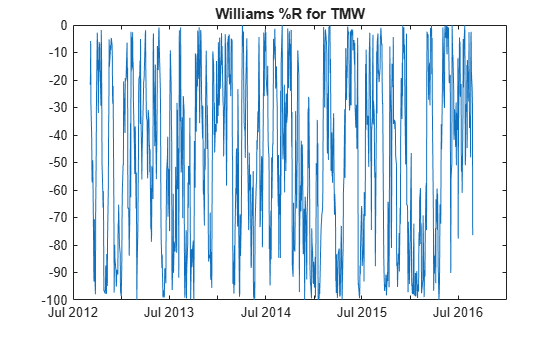# willpctr

Using a `fints` object for the `Data` argument of `willpctr` is not recommended. Use a matrix, `timetable`, or `table` instead for financial time series. For more information, see Convert Financial Time Series Objects fints to Timetables.

## Syntax

``PercentR = willpctr(Data)``
``PercentR = willpctr(___,Name,Value)``

## Description

example

````PercentR = willpctr(Data)` calculates the Williams PercentR (%R) values for a data series of with high, low, and closing prices.```

example

````PercentR = willpctr(___,Name,Value)` adds optional name-value pair arguments. ```

## Examples

collapse all

Load the file `SimulatedStock.mat`, which provides a timetable (`TMW`) for financial data for TMW stock.

```load SimulatedStock.mat PercentR = willpctr(TMW); plot(PercentR.Time,PercentR.WillPercentR) title('Williams %R for TMW')```## Input Arguments

collapse all

Data with high, low, open, close information, specified as a matrix, table, or timetable. For matrix input, `Data` is an `M`-by-`3` with high, low, and closing prices stored in the corresponding columns. Timetables and tables with `M` rows must contain variables named `'High'`, `'Low'`, and `'Close'` (case insensitive).

Data Types: `double` | `table` | `timetable`

### Name-Value Arguments

Specify optional pairs of arguments as `Name1=Value1,...,NameN=ValueN`, where `Name` is the argument name and `Value` is the corresponding value. Name-value arguments must appear after other arguments, but the order of the pairs does not matter.

Before R2021a, use commas to separate each name and value, and enclose `Name` in quotes.

Example: `PercentR = willpctr(TMW,'NumPeriods',15)`

Moving window for Williams PercentR, specified as the comma-separated pair consisting of `'NumPeriods'` and a scalar positive integer.

Data Types: `double`

## Output Arguments

collapse all

Williams PercentR series, returned with the same number of rows (`M`) and the same type (matrix, table, or timetable) as the input `Data`.